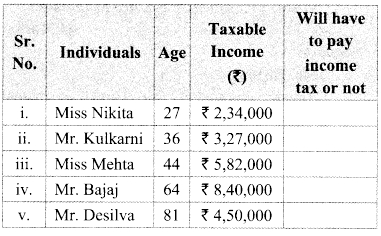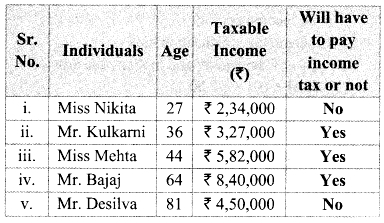# Maharashtra Board 9th Class Maths Part 1 Practice Set 6.2 Solutions Chapter 6 Financial Planning

Balbharti Maharashtra State Board Class 9 Maths Solutions covers the Practice Set 6.2 Algebra 9th Class Maths Part 1 Answers Solutions Chapter 6 Financial Planning.

## Practice Set 6.2 Algebra 9th Std Maths Part 1 Answers Chapter 6 Financial Planning

Question 1.
Observe the table given below. Check and decide, whether the individuals have to pay income tax.Solution:
i. Miss Nikita’s age = 27 years < 60 years
Miss Nikita’s income = ₹ 2,34,000
Miss Nikita’s income is below the basic
exemption limit of ₹ 2,50,000.
∴ Miss Nikita will not have to pay income tax.

ii. Mr. Kulkarni’s age 36 years < 60 years
Mr. Kulkarni’s income = ₹3,27,000
Mr. Kulkarni’s income is above the basic exemption Limit of ₹2,50,000.
∴ Mr. Kulkarni will have to pay income tax.

iii. Miss Mehta’s age = 44 years < 60 years Miss Mehta’s income = ₹5.82,000
Miss Mehta’s income is above the basic exemption limit of ₹2,50,000.
∴ Miss Mehta will have to pay income tax.

iv. Mr. Bajaj’s age = 64 years (Age 60 to 80 years)
Mr. Bajaj’s income = ₹8,40,000
Mr. Bajaj’s income is above the basic exemption Limit of ₹3,00,000.
∴ Mr. Bajaj will have to pay income tax.

v. Mr. Desilva’s age = 81 years > 80 years
Mr. Desilva’s income = ₹4,50,000
Mr. Desilva’s income is below the basic exemption limit of ₹ 5,00.000.
∴ Mr. Desilva will not have to pay income tax.Question 2.
Mr. Kartarsingh (age 48 years) works in a private company. His monthly income after deduction of allowances is ₹ 42,000 and every month he contributes ₹ 3000 to GPF. He has also bought ₹ 15,000 worth of NSC (National Savings Certificate) and donated ₹ 12,000 to the PM’s Relief Fund. Compute his income tax.
Solution:
Mr. Kartarsingh’s monthly income = ₹ 42,000
Mr. Kartarsingh’s yearly income = 42,000 x 12 = ₹ 5,04,000

Mr. Kartarsingh’s investment
= GPF + NSC
= (3000 x 12)+ 15,000
= 36,000 + 15,000
= ₹ 51,000

Donation to PM’s relief fund = ₹ 12, 000
∴ Taxable income
= yearly income – (investment + donation)
= 5,04,000 – (51,000 + 12,000)
= 5,04,000 – 63,000 = ₹ 4,41,000
Mr. Kartarsingh income falls in the slab 2,50,001 to 5,00,000.
∴ Income tax = 5% of (Taxable income – 250000) = 5% of (4,41,000 – 2,50,000)
= $$\frac { 5 }{ 100 }$$ x 1,91,000 100
= ₹ 9550
Education cess = 2% of income tax
= $$\frac { 2 }{ 100 }$$ x 9550
= 191
Secondary and Higher Education cess = 1% of income tax
= $$\frac { 1 }{ 100 }$$ x 9550 100
= 95.50
Total income tax = Income tax + Education cess + Secondary and higher education cess
= 9550 + 191 + 95.50
= ₹ 9836.50
∴ Mr. Kartarsingh’s income tax is ₹ 9836.50

Maharashtra Board Class 9 Maths Chapter 6 Financial Planning Practice Set 6.2 Intext Questions and Activities

Question 1.
Use Table I given above and write the appropriate amount/figure in the boxes for the example given below. (Textbook pg. no. 102)
Mr. Mehta’s annual income is ₹4,50,000
i. If he does not have any savings by which he can claim deductions from his income, to which slab does his taxable income belong ? ______
ii, What is the amount on which he will have to pay income tax and at what percent rate? on ₹ _______
percentage _______
iii. On what amount will the cess be levied? _______
1. ₹2,50,001 to ₹5,00,000
ii. 5% of (4,50,000 – 2,50,000)
i.e. 5% of ₹2,00,000
iii. income tax = 5% of 2,00,000
= $$\frac { 5 }{ 100 }$$ x 2,00,000
= ₹10,000
∴ Education cess and Secondary and higher education cess will be levied on the income tax i.e., on ₹10,000.

Question 2.
Use table lito carry out the following activity.
Mr. Pandit is 75 years old. Last year his annual income was ₹ 13,25,000. How much is his taxable income? How much tax does he have to pay? (Textbook pg. no. 103)
Solution:
Mr. Pandit’s age = 75 years (Age 60 to 80 years)
Mr. Pandit’s income is more than 10,00,000.
According to the table,
Income tax = ₹ 1,10,000 + 30 % of (taxable income – 10,00,000)
Taxable income – 10,00,000 = 13,25,000 – 10,00,000 = 3,25,000
In addition, on ₹ 3,25,000 rupees he has to pay 30% income tax.
3,25,000 x $$\frac { 30 }{ 100 }$$ = ₹ 97500
Therefore, his total income tax amounts to 1,10,000 + 97,500 ₹ 207500
Besides this, education cess willi be 2% of income tax 207500 x $$\frac { 2 }{ 100 }$$ = ₹ 4150.
A secondary and higher education cess at 1% of income tax = 207500 x $$\frac { 1 }{ 100 }$$ = ₹ 2075.
∴ Total income tax = Income tax + education cess + secondary and higher education cess
= 207500 + 4150 + 2075
= ₹2,13,725